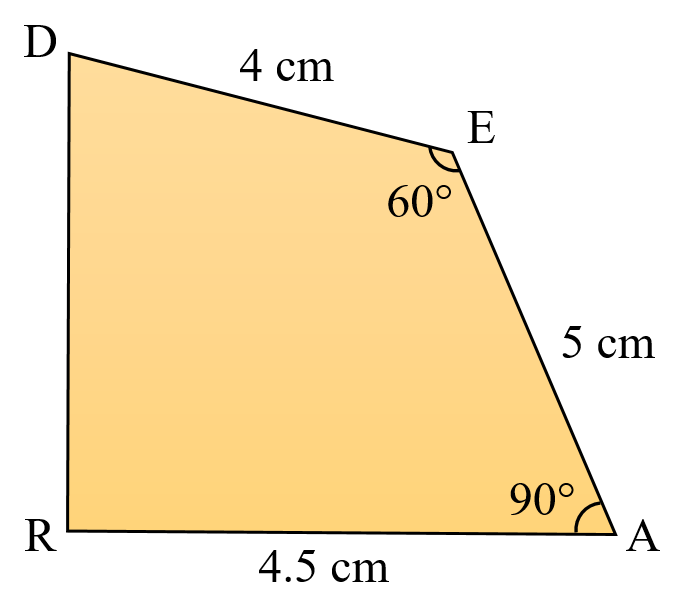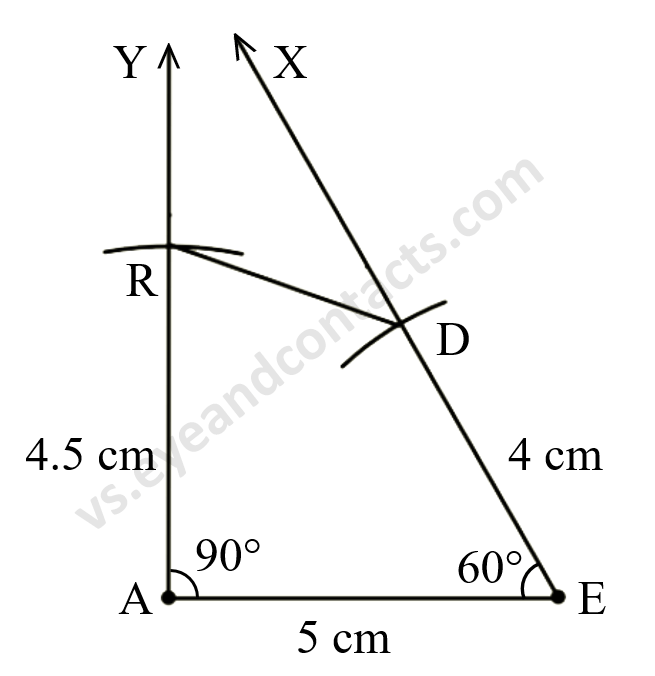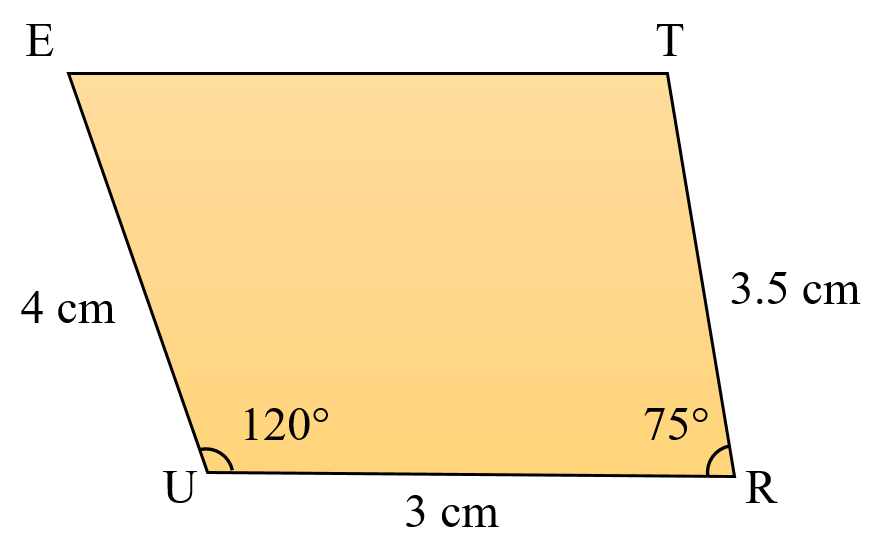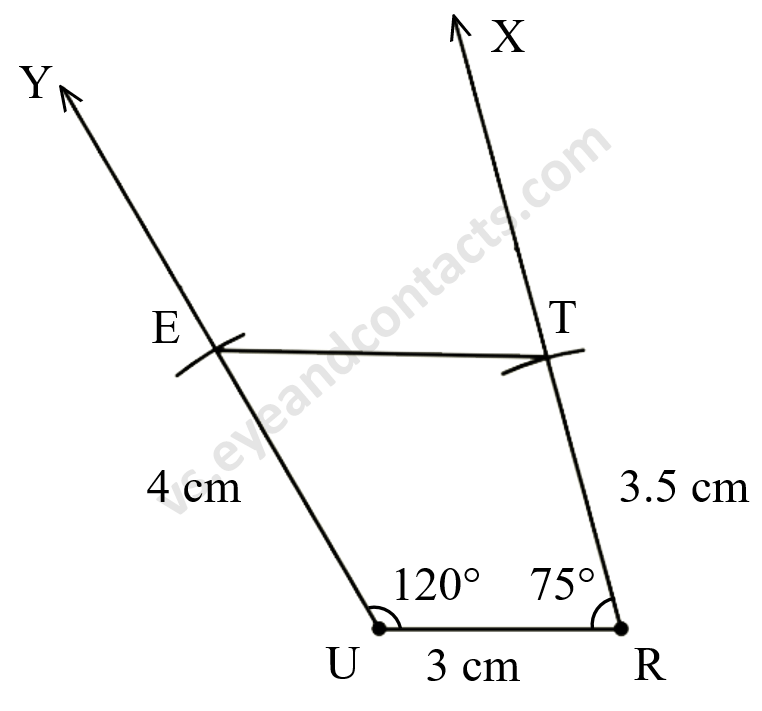# vs.eyeandcontacts.com

## Chapter 4 Practical Geometry Exercise 4.4

Question 1: Construct the following quadrilaterals.
DE = 4 cm
EA = 5 cm
AR = 4.5 cm
∠E = 60°
∠A = 90°
TR = 3.5 cm
RU = 3 cm
UE = 4 cm
∠R = 75°
∠U = 120°

i)
Given
DE = 4 cm
EA = 5 cm
AR = 4.5 cm
∠E = 60°
∠A = 90°

Rough figureActual figureSteps of Construction
1. Draw line segment AE = 5 cm.
2. Make ∠YEA= 90°.
3. Make ∠XEA = 60°.
4. With A as centre, radius 4.5 cm draw an arc to cut ray AY at R.
5. With E as centre radius 4 cm draw an arc to cut ray EX at D.
6. Join R and D.
7. DEAR is the required quadrilateral.

ii)
Given
TR = 3.5 cm
RU = 3 cm
UE = 4 cm
∠R = 75°
∠U = 120°

Rough figureActual figureSteps of Construction
1. Draw line segment UR = 3 cm.
2. Make ∠YUR = 120°.
3. Make ∠XRU = 75°.
4. With U as centre, radius 4 cm draw an arc to cut ray YU at E.
5. With R as centre, radius 3.5 cm draw an arc to cut ray XR at T.
6. Join E and T.
7. TRUE is the required quadrilateral.